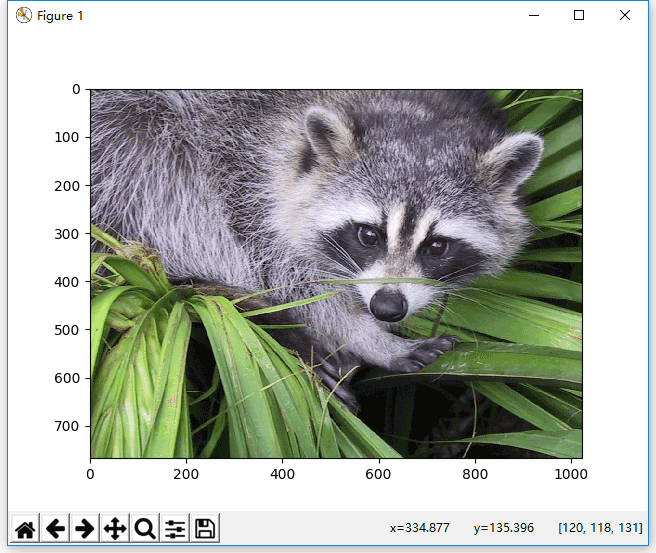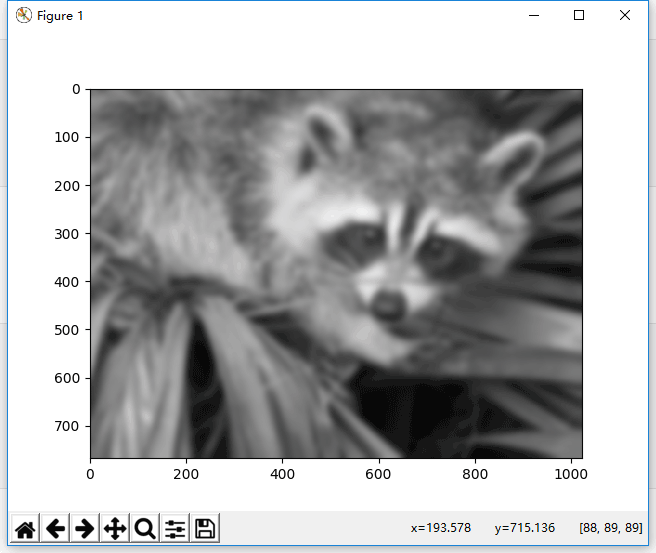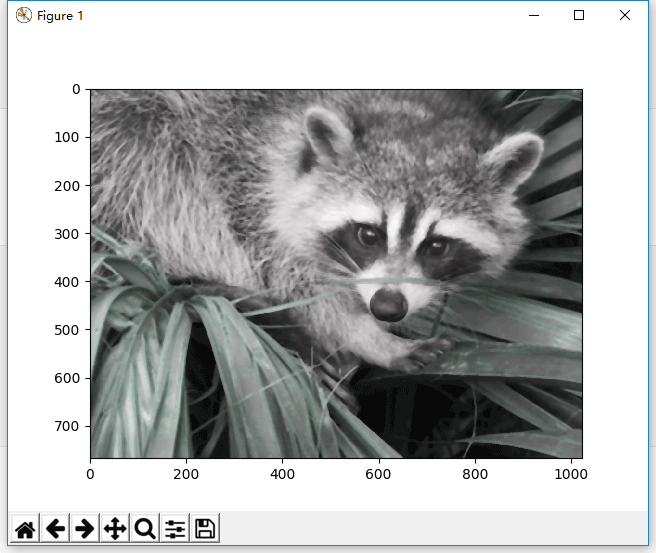# Python图像处理模块ndimage用法实例分析

更新时间：2019年09月05日 11:57:25   作者：cakincqm我要评论

1 代码

```from scipy import misc
from scipy import ndimage
import matplotlib.pyplot as plt
face = misc.face()#face是测试图像之一
plt.figure()#创建图形
plt.imshow(face)#绘制测试图像
plt.show()#原始图像

```

2 运行结果1 代码

```from scipy import misc
from scipy import ndimage
import matplotlib.pyplot as plt
face = misc.face()#face是测试图像之一
plt.figure()#创建图形
blurred_face = ndimage.gaussian_filter(face, sigma=7)#高斯滤波
plt.imshow(blurred_face)
plt.show()

```

2 运行结果1 代码

```from scipy import misc
from scipy import ndimage
import matplotlib.pyplot as plt
face = misc.face()#face是测试图像之一
plt.figure()#创建图形
blurred_face1 = ndimage.gaussian_filter(face, sigma=1)#边缘锐化
blurred_face3 = ndimage.gaussian_filter(face, sigma=3)
sharp_face = blurred_face3 +6*(blurred_face3-blurred_face1)
plt.imshow(sharp_face)
plt.show()

```

2 运行结果1 代码

```from scipy import misc
from scipy import ndimage
import matplotlib.pyplot as plt
face = misc.face()#face是测试图像之一
plt.figure()#创建图形
median_face = ndimage.median_filter(face,7)#中值滤波
plt.imshow(median_face)
plt.show()

```

2 运行结果